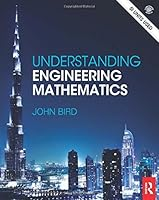# Understanding Engineering Mathematics0 Reviews
2014-02-06
1184 pages

## Book Description

Studying , whether it is mechanical, electrical or civil relies heavily on an understanding of . This new textbook clearly demonstrates the relevance of mathematical principles and shows how to apply them to solve real-life problems.

It deliberately starts at an elementary level so that students who are starting from a low knowledge base will be able to quickly get up to the level required. Students who have not studied mathematics for some time will find this an excellent refresher.

Each chapter starts with the basics before gently increasing in complexity. A full outline of essential definitions, formulae, laws and procedures are introduced before real world situations, practicals and problem solving demonstrate how the theory is applied.

Focusing on learning through practice, it contains examples, supported by 1,600 worked problems and 3,000 further problems contained within exercises throughout the text. In addition, 34 revision tests are included at regular intervals.

An interactive companion website is also provided containing 2,750 further problems with worked solutions and instructor materials

Section A Number and Algebra
1 Basic arithmetic
2 Fractions
3 Decimals
4 Using a calculator
5 Percentages
6 Ratio and proportion
7 Powers, roots and laws of indices
8 Units, prefixes and engineering notation
9 Basic algebra
10 Further algebra
11 Solving simple equations
12 Transposing formulae
13 Solving simultaneous equations
15 Logarithms
16 Exponential functions
17 Inequalities

Section B Further number and algebra
18 Polynomial division and the factor and remainder theorems
19 Number sequences
20 Binary, octal and hexadecimal numbers
21 Partial fractions
22 The binomial series
23 Maclaurin’s series
24 Hyperbolic functions
25 Solving equations by iterative methods
26 Boolean algebra and circuits

Section C Areas and volumes
27 Areas of common shapes
28 The circle and its properties
29 Volumes and surface areas of common solids
30 Irregular areas and volumes, and mean values

Section D
31 Straight line graphs
32 Graphs reducing non-linear laws to linear form
33 Graphs with logarithmic scales
34 Polar curves
35 Graphical solution of equations
36 Functions and their curves

Section E Geometry and trigonometry
37 Angles and triangles
38 Introduction to trigonometry
39 Trigonometric waveforms
40 Cartesian and polar co-ordinates
41 Non-right-angled triangles and some practical applications
42 Trigonometric identities and equations
43 The relationship between trigonometric and hyperbolic functions
44 Compound angles

Section F Complex numbers
45 Complex numbers
46 De Moivre’s theorem

Section G Matrices and determinants
47 The theory of matrices and determinants
48 Applications of matrices and determinants

Section H Vector geometry
49 Vectors
50 Methods of adding alternating waveforms
51 Scalar and vector products

Section I Differential calculus
52 Introduction to differentiation
53 Methods of differentiation
54 Some applications of differentiation
55 Differentiation of parametric equations
56 Differentiation of implicit functions
57 Logarithmic differentiation
58 Differentiation of hyperbolic functions
59 Differentiation of inverse trigonometric and hyperbolic functions
60 Partial differentiation
61 Total differential, rates of change and small changes
62 Maxima, minima and saddle points for functions of two variables

Section J Integral calculus
63 Standard
64 Integration using algebraic substitutions
65 Integration using trigonometric and hyperbolic substitutions
66 Integration using partial fractions
67 The t=tanθ/2 substitution
68 Integration by parts
69 Reduction formulae
70 Double and triple integrals
71 Numerical integration
72 Areas under and between curves
73 Mean and root mean square values
74 Volumes of solids of revolution
75 Centroids of simple shapes
76 Second moments of area

Section K Differential equations
77 Solution of first-order differential equations by separation of variables
78 Homogeneous first-order differential equations
79 Linear first-order differential equations
80 Numerical methods for first-order differential equations
81 Second-order differential equations of the form a d2y/dx2+bdy/dx+cy=0
82 Second-order differential equations of the form ad2y/dx2+bdy/dx+cy=f(x)
83 Power seriesmethods of solving ordinary differential equations
84 An introduction to partial differential equations

Section L Statistics and probability
85 Presentation of statistical data
86 Mean, median, mode and standard deviation
87 Probability
88 The binomial and Poisson distributions
89 The normal
90 Linear correlation
91 Linear regression
92 Sampling and estimation theories
93 Significance testing
94 Chi-square and distribution-free tests

Section M Laplace transforms
95 Introduction to Laplace transforms
96 Properties of Laplace transforms
97 Inverse Laplace transforms
98 The Laplace transform of the Heaviside function
99 The solution of differential equations using Laplace transforms
100 The solution of simultaneous differential equations using Laplace transforms

Section N Fourier series
101 Fourier series for periodic functions of period 2π
102 Fourier series for a non-periodic function over range 2π
103 Even and odd functions and half-range Fourier series
104 Fourier series over any range
105 A numerical method of harmonic analysis
106 The complex or exponential form of a Fourier series

## Book Details

• Title: Understanding Engineering Mathematics
• Author:
• Length: 1184 pages
• Edition: 1
• Language: English
• Publisher:
• Publication Date: 2014-02-06
• ISBN-10: 0415662842
• ISBN-13: 9780415662840